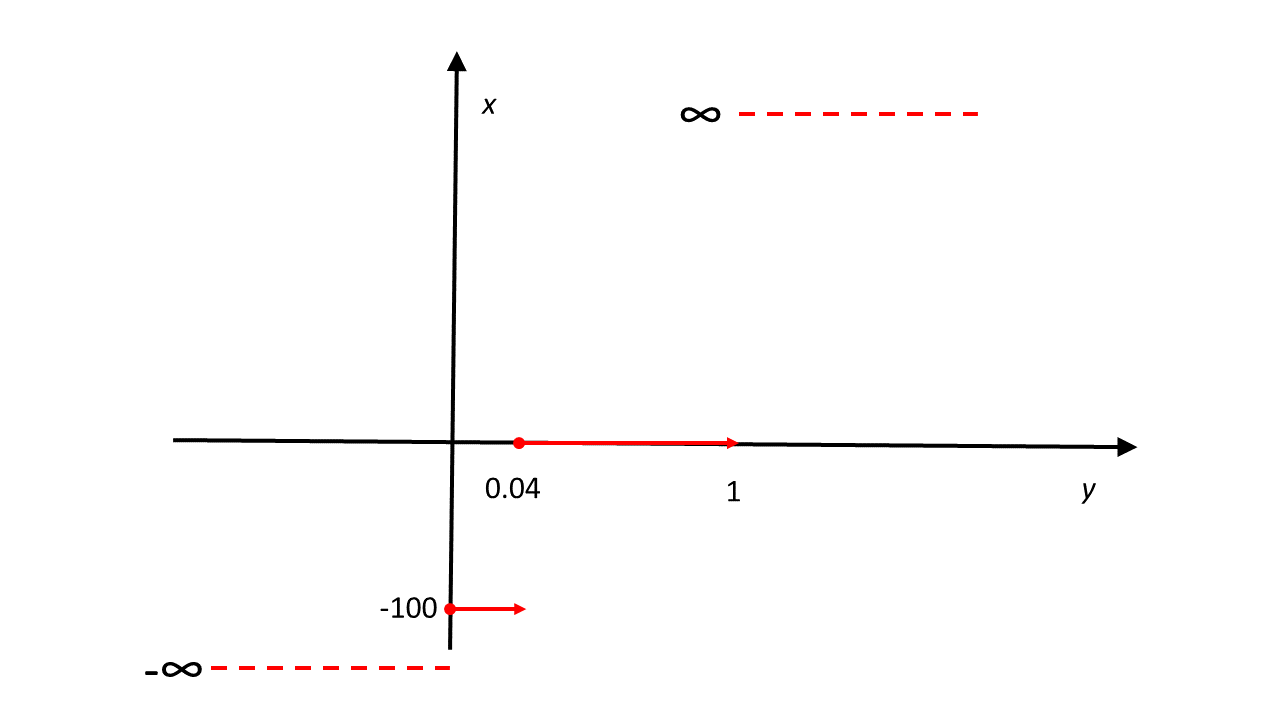7
May 19

## Question 1 from UoL exam 2016, Zone B, Post 1## Question 1 from UoL exam 2016, Zone B, Post 1

There is a hidden mine in this question, and it is caused by discreteness of the distribution function. We had a lively discussion of this oddity in my class. The answer will be given in two posts.

Question. Two corporations each have a 4% chance of going bankrupt and the event that one of the two companies will go bankrupt is independent of the event that the other company will go bankrupt. Each company has outstanding bonds. A bond from any of the two companies will return$R=0%$ if the corporation does not go bankrupt, and if it goes bankrupt you lose the face value of the investment, i.e.,$R=-100%$. Suppose an investor buys $1000 worth of bonds of the first corporation, which is then called portfolio$P_1$, and similarly, an investor buys$1000 worth of bonds of the second corporation, which is then called portfolio$P_2$.

(a) [40 marks] Calculate the VaR at$\alpha=5\%$ critical level for each portfolio and for the joint portfolio$P_1+P_2$.

(b) [30 marks] Is VaR sub-additive in this example? Explain why the absence of sub-additivity may be a concern for risk managers.

(c) [30 marks] The expected shortfall$ES^\alpha$ at the$\alpha =5\%$ critical level can be defined as$ES^\alpha=-E_t[R|R<-VaR_{t+1}^\alpha]$. Calculate the expected shortfall for the portfolios$P_1$,$P_2$ and$P_1+P_2$. Is this risk measure sub-additive?

Solution. a) The return on each portfolio is a binary variable described by Table 1:

Table 1. Return on separate portfolios$R_i$$R_i$ Prob 0 0.96 -100 0.04

Therefore the distribution function$F_{R_i}(x)$ of the return is a piece-wise constant function equal to$0$ for$x<-100,$ to$0.04$ for$-100\leq x<0$ and to$1$ for$x\geq 0,$ see Example 3. For instance, if$x\geq 0$ we can write$F_{R_i}(x)=P(R_i\leq x)=P(R_i<-100)+P(R_i=-100)+P(-100Diagram 1. Return distribution function

Since this function is not one-to-one, it's usual inverse does not exist and we have to use the quasi-inverse, see Answer 15. As with the distribution function, we need to look at different cases.

If$y>1,$ drawing a horizontal line at$y$ we see that the set$\{x:F_{R_i}(x)\geq y\}$ is empty and the infimum of an empty set is by definition$+\infty$ (can you guess why?)

For any$1\geq y>0.04,$ we have$\{x:F_{R_i}(x)\geq y\}=[0,+\infty ),$ so$F_{R_i}^{-1}(y)=0.$

Next, for$0 we have$\{x:F_{R_i}(x)\geq y\}=[-100,+\infty )$ and$F_{R_i}^{-1}(y)=-100.$

Finally, if$y\leq 0,$ we get$\{x:F_{R_i}(x)\geq y\}=(-\infty ,+\infty )$ and$F_{R_i}^{-1}(y)=-\infty .$Generalized inverse example

The resulting function is bad in two ways. Firstly, it takes infinite values. In applications to VaR this should not concern us because the bad values occur in the ranges$y>1$ and$y\leq 0.$

Secondly, for practically interesting values of$y\in (0,1),$ the graph of$F_{R_i}^{-1}$ has flat pieces, which may be problematic. By definition,$VaR^\alpha$ is the solution to the equation$P(R_i\leq VaR^\alpha)=\alpha .$

This means that we should have$VaR^\alpha=F_{R_i}^{-1}(\alpha).$ When we plug this value in$F_{R_i}(x),$ we are supposed to get$\alpha .$ However, here we don't, in general. For example,$VaR^{0.02}=F_{R_i}^{-1}(0.02)=-100,$ while$F_{R_{i}}(-100)=0.04\neq 0.02.$

This happens because the usual inverse does not exist. When the usual inverse exists, we have two identities$F^{-1}(F(x))=x$ and$F(F^{-1}(y))=y.$ Here both are violated.

Now the definition of VaR gives$VaR_{R_i}^\alpha=F_{R_i}^{-1}(0.05)=0$ for each portfolio.